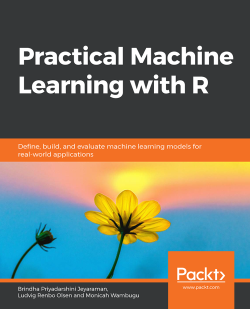# Practical Machine Learning with R

By Brindha Priyadarshini Jeyaraman , Ludvig Renbo Olsen , Monicah Wambugu
• \$25.99 eBook
• \$36.99 Print + eBook
• \$12.99 eBook + Subscription
What do you get with a Packt Subscription?

• Constantly updated with 100+ new titles each month
• Breadth and depth in over 1,000+ technologies

With huge amounts of data being generated every moment, businesses need applications that apply complex mathematical calculations to data repeatedly and at speed. With machine learning techniques and R, you can easily develop these kinds of applications in an efficient way.

Practical Machine Learning with R begins by helping you grasp the basics of machine learning methods, while also highlighting how and why they work. You will understand how to get these algorithms to work in practice, rather than focusing on mathematical derivations. As you progress from one chapter to another, you will gain hands-on experience of building a machine learning solution in R. Next, using R packages such as rpart, random forest, and multiple imputation by chained equations (MICE), you will learn to implement algorithms including neural net classifier, decision trees, and linear and non-linear regression. As you progress through the book, you’ll delve into various machine learning techniques for both supervised and unsupervised learning approaches. In addition to this, you’ll gain insights into partitioning the datasets and mechanisms to evaluate the results from each model and be able to compare them.

By the end of this book, you will have gained expertise in solving your business problems, starting by forming a good problem statement, selecting the most appropriate model to solve your problem, and then ensuring that you do not overtrain it.

Publication date:
August 2019
Publisher
Packt
Pages
416
ISBN
9781838550134

# An Introduction to Machine Learning

## Learning Objectives

By the end of this chapter, you will be able to:

• Explain the concept of machine learning.
• Outline the process involved in building models in machine learning.
• Identify the various algorithms available in machine learning.
• Identify the applications of machine learning.
• Use the R command to load R packages.
• Perform exploratory data analysis and visualize the datasets.

This chapter explains the concept of machine learning and the series of steps involved in analyzing the data to prepare it for building a machine learning model.

## Introduction

Machine learning is a process through which we use data to train models. These models are then used to make predictions on a new set of data that the model hasn't seen before. There are different types of machine learning models, such as supervised learning, unsupervised learning, semi-supervised learning, and reinforcement learning.

The data in a supervised learning model should have labels or an end result. Supervised learning models are broadly classified into classification and regression learning models. In an unsupervised learning process, we may not know the labels or the outcomes beforehand. Clustering is an example of unsupervised learning. Semi-supervised learning models use a combination of supervised and unsupervised learning. In reinforcement learning, an agent learns to navigate an environment with feedback mechanisms that reinforce the goal maximizing actions.

In this chapter, the machine learning process will be demonstrated through examples. The types of machine learning models will be explained and the different evaluation metrics are discussed. We will learn to perform exploratory data analysis and implement a simple linear model in R.

## The Machine Learning Process

The machine learning process is a sequence of activities performed to deploy a successful model for prediction. A few steps here are iterative and can be repeated based on the outcomes of the previous and the following steps. To train a good model, we must clean our data and understand it well from the business perspective. Using our understanding, we can generate appropriate features. The performance of the model depends on the goodness of the features.

A sample model building process follows these steps: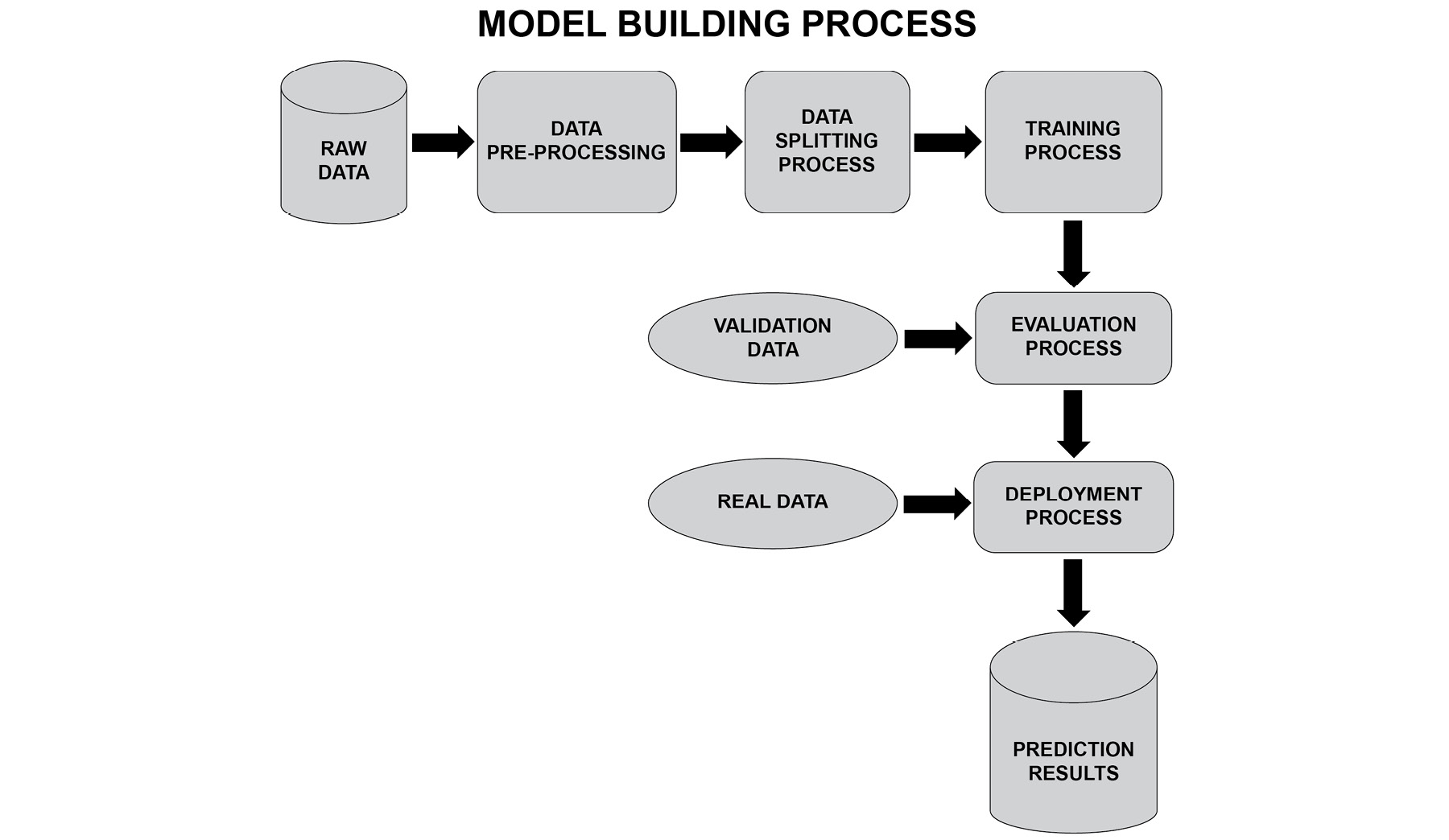###### Figure 1.1: The model building process

The model building process consists of obtaining the right data, processing it to derive features, and then choosing the right model. Based on the type of output to be predicted, we need to choose a model. For example, if the output is categorical, a decision tree can be chosen; if the output is numerical, a neural network can be chosen. However, decision trees also can be used for regression and neural networks can be used for classification. Choosing the right data means using data related to the output of our problem. For example, if we are predicting the value of a house, then information such as the location and size are all data that is highly correlated with the predicted value, hence it is of high value. Gathering and deriving good features can make a huge difference.

### Raw Data

The raw data refers to the unprocessed data that is relevant for the machine learning problem. For instance, if the problem statement is to predict the value of stocks, then the data constituting the characteristics of a stock and the company profile data may be relevant to the prediction of the stock value; therefore, this data is known as the raw data.

### Data Pre-Processing

The pre-processing step involves:

1. Data cleaning
2. Feature selection
3. Computing new features

The data cleaning step refers to activities such as handling missing values in the data, handling incorrect values, and so on. During the feature selection process, features that correlate with the output or otherwise are termed important are selected for the purpose of modeling. Additional meaningful features that have high correlation with the output to be predicted can also be derived; this helps to improve the model performance.

### The Data Splitting Process

The data can be split into an 80%-20% ratio, where 80% of the data is used to train the model and 20% of the data is used to test the model. The accuracy of the model on the test data is used as an indicator of the performance of the model.

Therefore, data is split as follows:

• Training data [80% of the data]: When we split our training data, we must ensure that 80% of the data has sufficient samples for the different scenarios we want to predict. For instance, if we are predicting whether it will rain or not, we usually want the training data to contain 40-60% of rows that represent will rain scenarios and 40-60% of rows that represent will not rain scenario.
• Testing data [20% of the data]: 20% of the data reserved for testing purposes must have a sample for the different cases/classes we are predicting for.
• Validation data [new data]: The validation data is any unseen data that is passed to the model for prediction. The measure of error on the validation data is also an indicator of the performance of the model.

### The Training Process

The training phase involves the following:

1. Selecting a model
2. Training the model
3. Measuring the performance
4. Tuning the model

A machine learning model is selected for the purpose of training. The performance of the model is measured in the form of precision, recall, a confusion matrix, and so on. The performance is analyzed, and the parameters of the model are tuned to improve the performance.

### Evaluation Process

The following are some of the metrics used for the evaluation of a machine learning model:

• Accuracy

Accuracy is defined as the % of correct predictions made.

Accuracy = Number of correct predictions/Total number of predictions

• Confusion matrix

Let's consider a classification problem that has an output value of either positive or negative. The confusion matrix provides four types of statistics in the form of a matrix for this classification problem.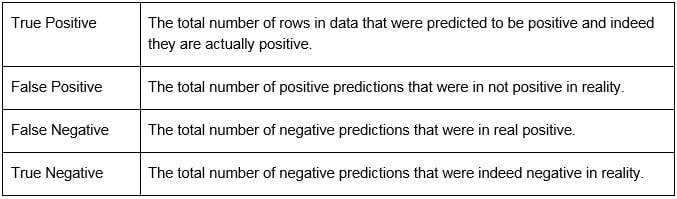###### Figure 1.2: Statistics in a classification problem

Let's take an example of patients diagnosed with diabetes. Here, the model has been trained to predict whether a patient has diabetes or not. The actual class means the actual lab result for the person. The predicted class is the predicted result from the trained model.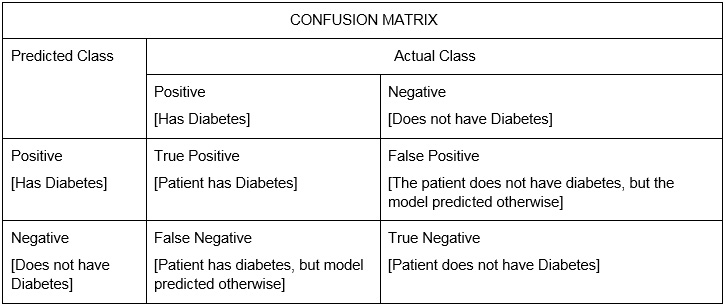###### Figure 1.3: A confusion matrix of diabetes data
• Precision

Precision = True positives/(True positives + False positives)

• Recall

Recall = True positives/(True positives + False negatives)

• Mean squared error

The difference between the original value and the predicted value is known as error. The average of the absolute square of the errors is known as the mean squared error.

• Mean absolute error

The difference between the original value and the predicted value is known as error. The average of the errors is known as the mean absolute error.

• RMSE

Root Mean Squared Error (RMSE) is the square root of the mean squared difference between the model predictions and the actual output values.

• ROC curve

The Receiver Operating Characteristic (ROC) curve is a visual measure of performance for a classification problem. The curve plots true positive rate to the false positive rate.

• R-squared

This measures the amount of variation in the output variable, which can be explained by the input variables. The greater the value, the more is the variation of output variable by the input variable.

This is used for multiple variable regression problems. It is similar to R-squared, but if the input variable added does does not improve the model's predictions, then the value of adjusted R-squared decreases.

### Deployment Process

This is also the prediction phase. This is the stage where the model with optimal performance is chosen for deployment. This model will then be used on real data. The performance of the model has to be monitored and the model has to be retrained at regular intervals if required so that the prediction can be made with better accuracy for the future data.

### Process Flow for Making Predictions

Imagine that you have to create a machine learning model to predict the value/price of the house by training a machine learning model. The process to do this is as follows:

1. Raw data: The input data should contain information about the house, such as its location, size, amenities, number of bedrooms, number of bathrooms, proximity to a train station, and proximity to a bus stop.
2. Data pre-processing: During the pre-processing step, we perform cleaning of the raw data; for example, handling missing values, removing outliers, and scaling the data. We then select the features from the raw data that are relevant for our house price prediction, such as location, proximity to a bus stop, and size. We would also create new features such as the amenity index, which is a weighted average of all the amenities like nearest supermarket, nearest train station, and nearest food court. This could be a value ranging from 0-1. We can also have a condition score, a combination of various factors to signify the move-in condition of the unit. Based on the physical appearance of the house, a subjective score can be given of between 0-1 for factors such as cleanliness, paint on walls, renovations done, and repairs to be done.
3. Data splitting: The data will be split into 80% for training and 20% for testing purposes.
4. Training: We can select any regression model, such as support vector regression, linear regression, and gradient boosted and implement them using R.
5. Evaluation: The models will be evaluated based on metrics such as, mean absolute error (MAE), and RMSE.
6. Deployment: The models will be compared with each other using the evaluation metrics. When the values are acceptable to us and the values do not overfit, we would proceed to deploy the model into production. This would require us to develop software to create a workflow for training, retraining, refreshing the models after retraining, and prediction on new data.

The process is now clear. Let's move on to R programming.

## Introduction to R

R provides an extensive set of libraries for visualization, data manipulation, statistical analysis, and model building. We will check the installation of R, perform some visualization, and build models in RStudio.

To test if the installation is successful, write this simple command as follows:

print("Hi")

The output is as follows:

"Hi"

After installing R, let's write the first R script in RStudio.

### Exercise 1: Reading from a CSV File in RStudio

In this exercise, we will set the working directory and then read from an existing CSV file:

1. We can set any directory containing all our code as the working directory so that we need not give the full path to access the data from that folder:

# Set the working directory

setwd("C:/R")

2. Write an R script to load data into data frames:

data

The output is as follows:

Col1 Col2 Col3

1    1    2    3

2    4    5    6

3    7    8    9

4    a    b    c

R scripts are simple to write. Let's move on to operations in R.

### Exercise 2: Performing Operations on a Dataframe

In this exercise, we will display the values of a column in the dataframe and also add a new column with values into the dataframe using the rbind() and cbind() functions.

1. Let's print Col1 values using the dataframe["ColumnName"] syntax:

data['Col1']

Col1

The output is as follows:

1    1

2    4

3    7

4    a

2. Create a new column Col4 using cbind() function. This is similar to rbind():

cbind(data,Col4=c(1,2,3,4))

The output is as follows:

Col1 Col2 Col3 Col4

1    1    2    3    1

2    4    5    6    2

3    7    8    9    3

4    a    b    c    4

3. Create a new row in the dataframe using the rbind() function:

rbind(data,list(1,2,3))

The output is as follows:

Col1 Col2 Col3

1    1    2    3

2    4    5    6

3    7    8    9

4    a    b    c

5    1    2    3

We have added columns to the dataframe using the rbind() and cbind() functions. We will move ahead to understanding how exploratory data analysis helps us understand the data better.

### Exploratory Data Analysis (EDA)

Exploratory Data Analysis (EDA) is the use of visualization techniques to explore the dataset. We will use the built-in dataset in R to learn to see a few statistics about the data. The datasets used are as follows: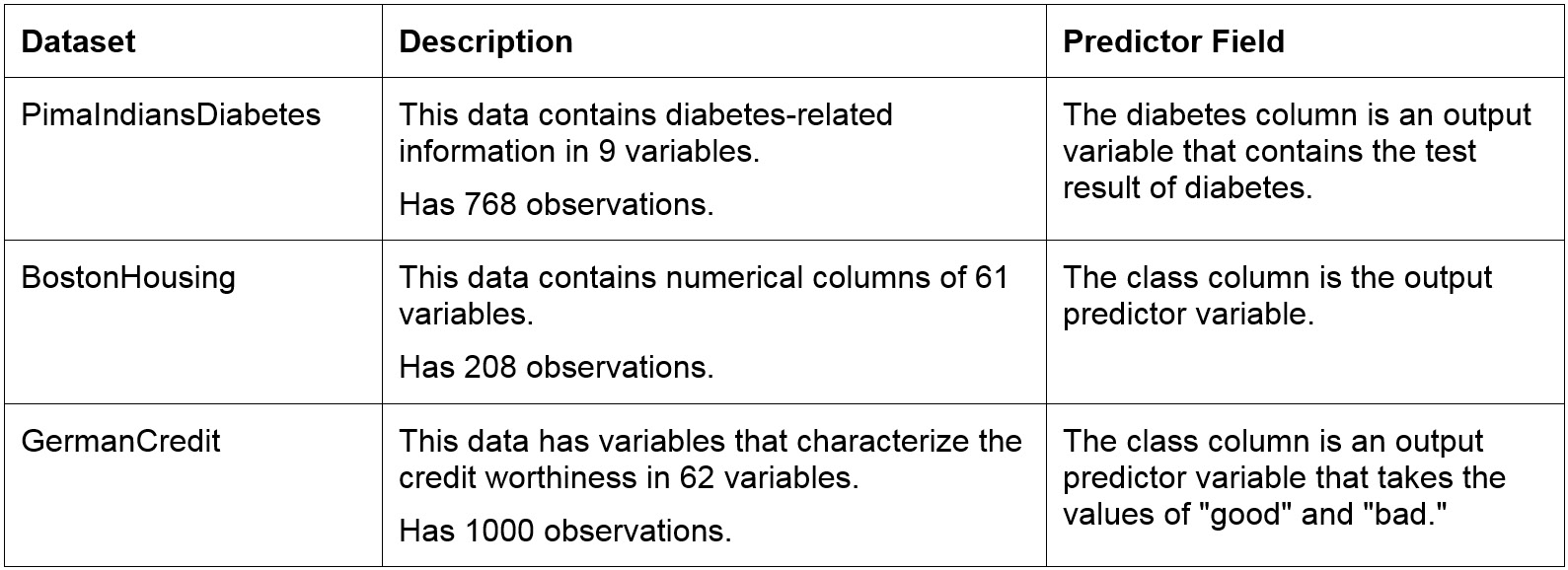### View Built-in Datasets in R

To install packages to R, we use the following syntax: install.packages("Name_of_package")

The pre-loaded datasets of R can be viewed using the data() command:

#Installing necessary packages

install.packages("mlbench")

install.packages("caret")

data(package = .packages(all.available = TRUE))

The datasets will be displayed in the dataset tab as follows: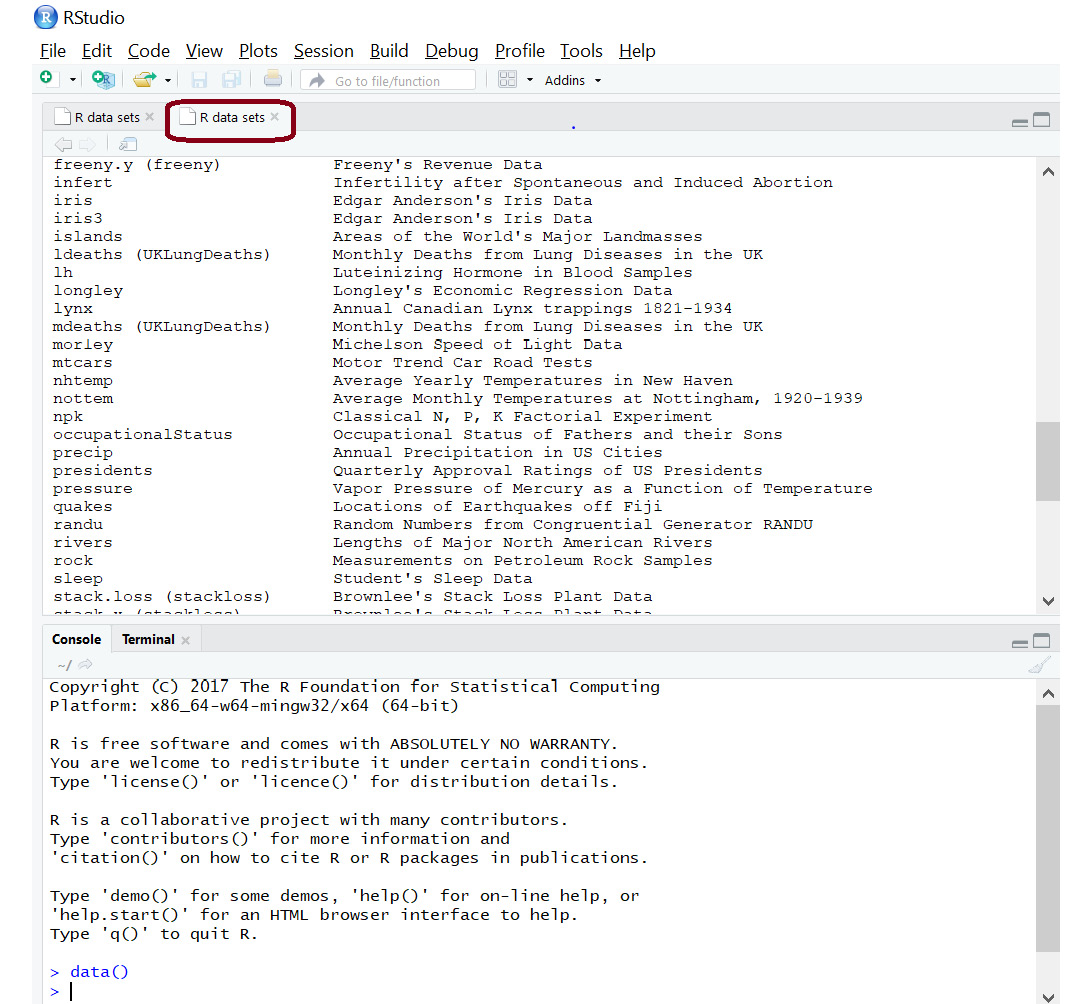###### Figure 1.5: Dataset tab for viewing all the datasets

We can thus install packages and load the built-in datasets.

In this exercise, we will load built-in datasets, analyze the contents of the datasets, and read the first and last records from those datasets.

1. We will use the BostonHousing and GermanCredit datasets shown in the following screenshot: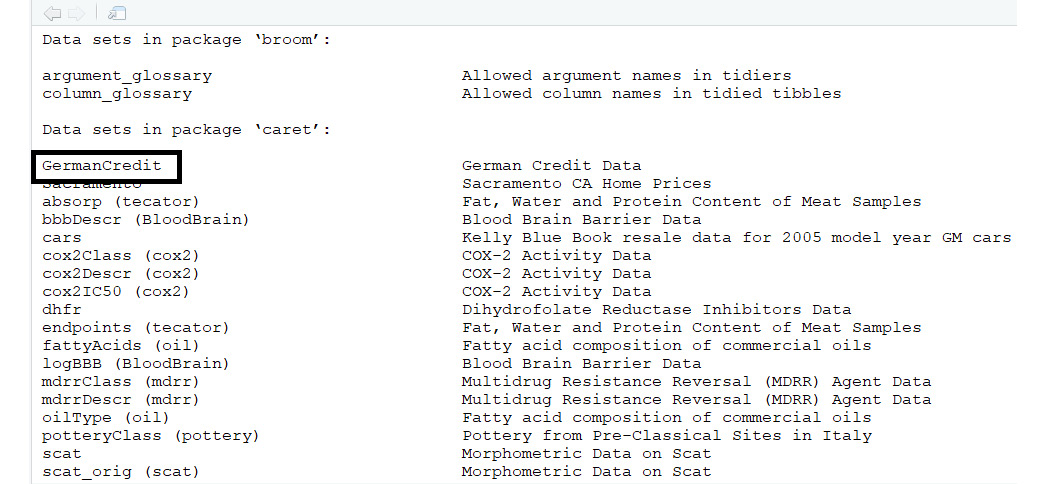###### Figure 1.6: The GermanCredit dataset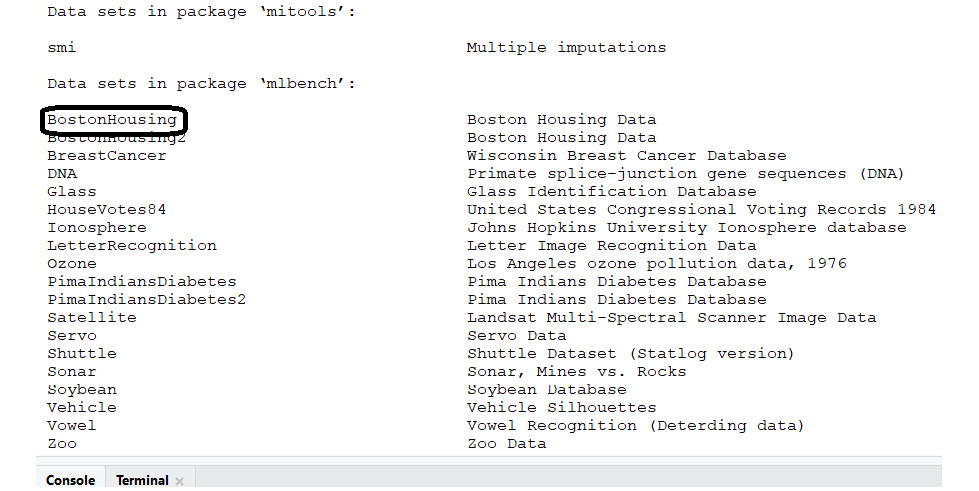###### Figure 1.7: The BostonHousing dataset
2. Check the installed packages using the following code:

data(package = .packages(all.available = TRUE))

3. Choose File | New File | R Script: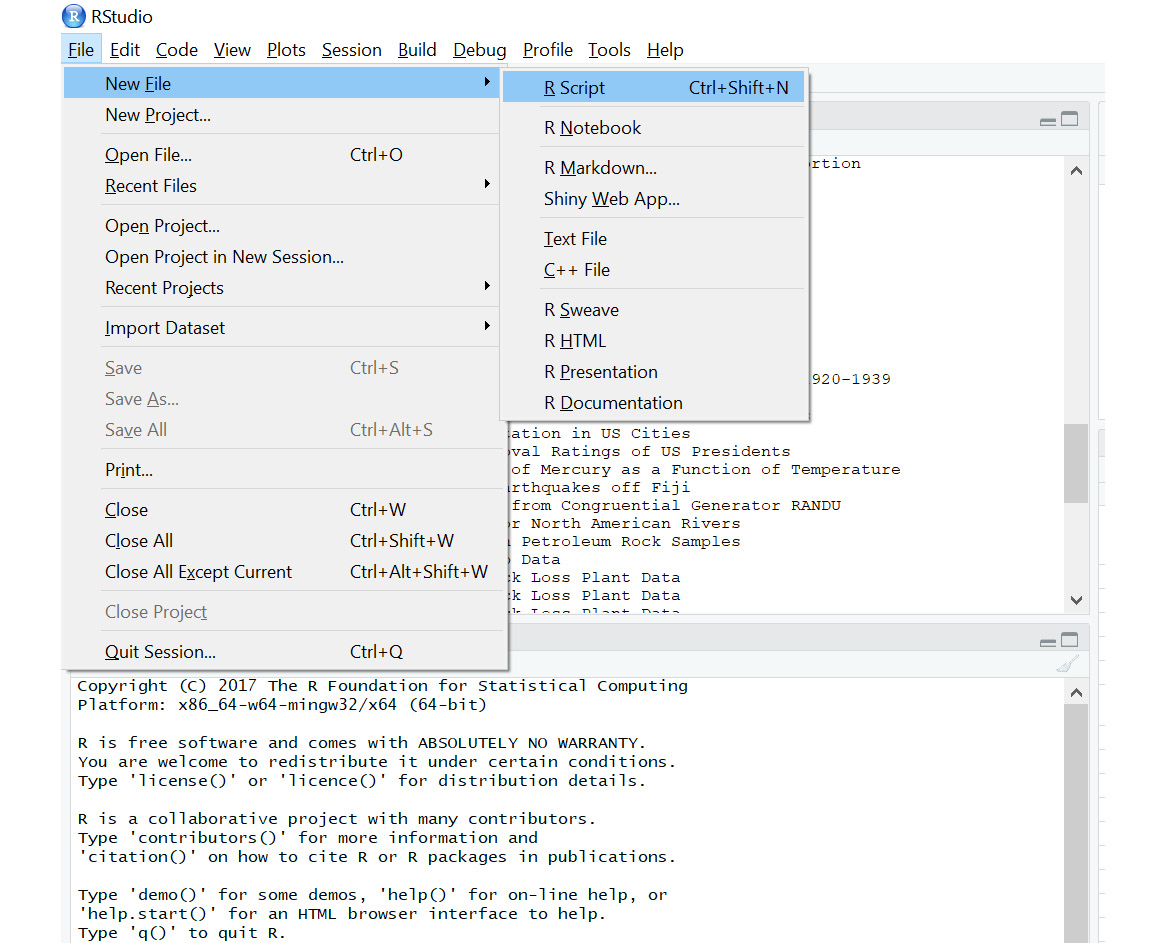###### Figure 1.8: A new R script window
4. Save the file into the local directory by clicking Ctrl + S on windows.
5. Load the mlbench library and the BostonHousing dataset:

library(mlbench)

data(BostonHousing)

6. The first five rows in the data can be viewed using the head() function, as follows:

#Print the first 5 lines in the dataset

7. Click the Run option as shown: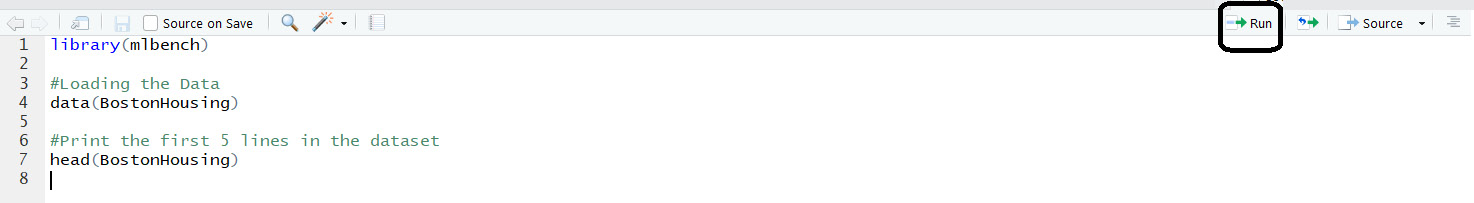###### Figure 1.9: The Run option

The output will be as follows: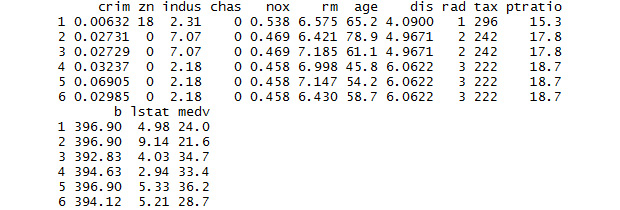###### Figure 1.10: The first rows of Boston Housing dataset
8. The description of the dataset can be viewed using <<Dataset>>. In place of <<Dataset>>, mention the name of the dataset:

# Display information about Boston Housing dataset

?BostonHousing

The Help tab will display all the information about the dataset. The description of the columns is available here: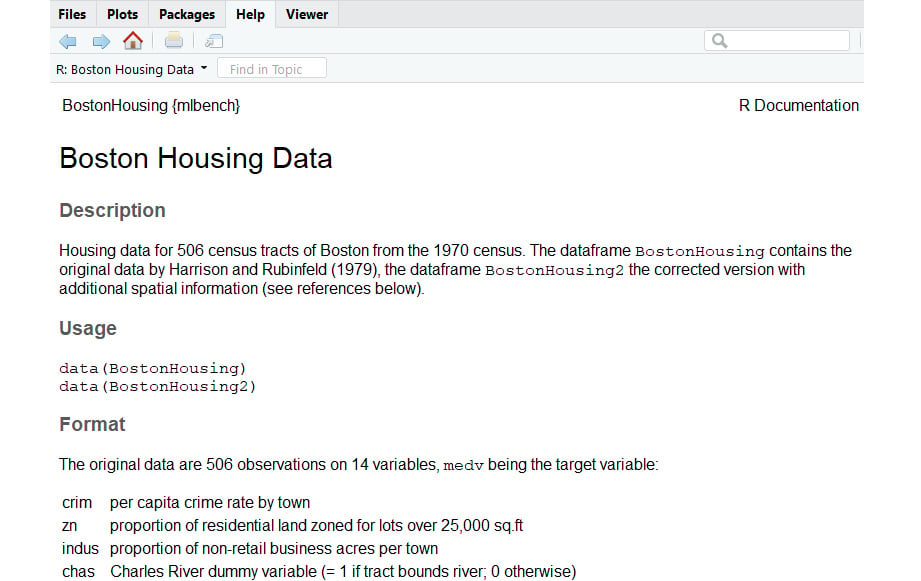9. The first n rows and last m rows in the data can be viewed as follows:

#Print the first 10 rows in the dataset

The output is as follows: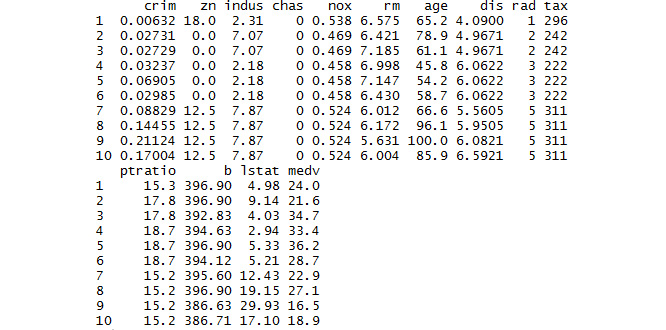###### Figure 1.12: The first 10 rows of the Boston Housing dataset
10. Print the last rows:

#Print the last rows in the dataset

tail(BostonHousing)

The output is as follows: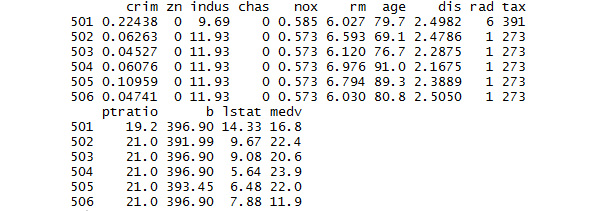###### Figure 1.13: The last rows of the Boston Housing dataset
11. Print the last 7 rows:

#Print the last 7 rows in the dataset

tail(BostonHousing,7)

The output is as follows: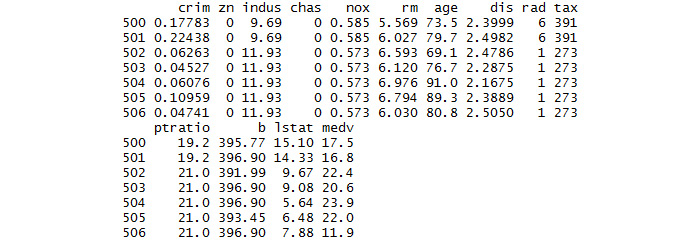###### Figure 1.14: The last seven rows of the Boston Housing dataset

Thus, we have loaded a built-in dataset and read the first and last lines from the loaded dataset. We have also checked the total number of rows and columns in the dataset by cross-checking it with the information in the description provided.

Selectively running lines of code:

We can select lines of code within the script and click the Run option to run only those lines of code and not run the entire script: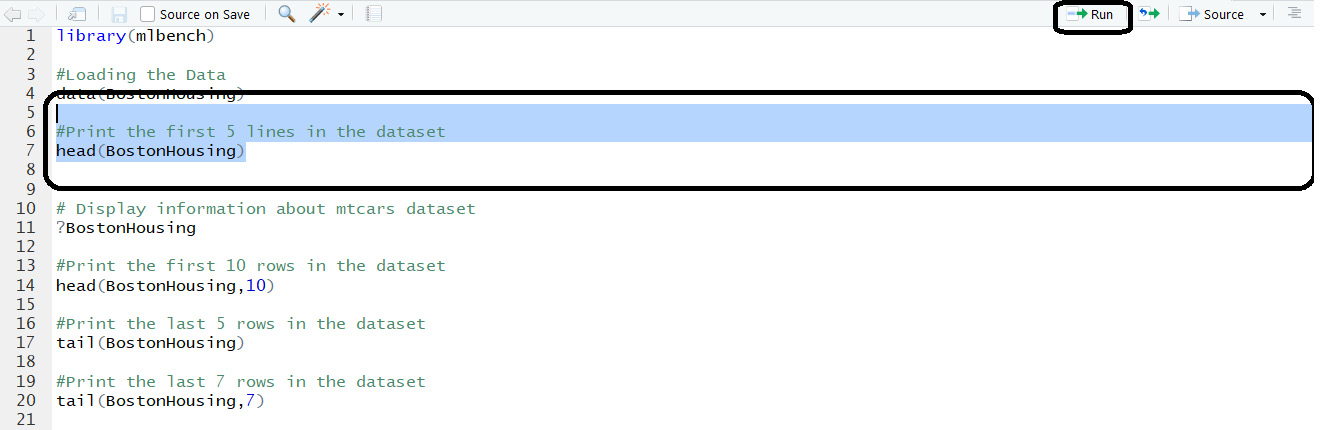###### Figure 1.15: Selectively running the code

Now, we will move to viewing a summary of the data.

### Exercise 4: Viewing Summaries of Data

To perform EDA, we need to know the data columns and data structure. In this exercise, we will cover the important functions that will help us explore data by finding the number of rows and columns in the data, the structure of the data, and the summary of the data.

1. The columns names of the dataset can be viewed using the names() function:

# Display column names of GermanCredit

library(caret)

data(GermanCredit)

# Display column names of GermanCredit

names(GermanCredit)

A section of the output is as follows: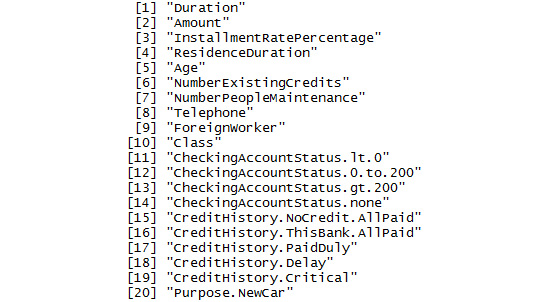###### Figure 1.16: A section of names in the GermanCredit dataset
2. The total number of rows in the data can be displayed using nrow:

# Display number of rows of GermanCredit

nrow(GermanCredit)

The output is as follows:

 1000

3. The total number of columns in the data can be displayed using ncol:

# Display number of columns of GermanCredit

ncol(GermanCredit)

The output is as follows:

 62

4. To know the structure of the data, use the str function:

# Display structure of GermanCredit

str(GermanCredit)

A section of the output is as follows: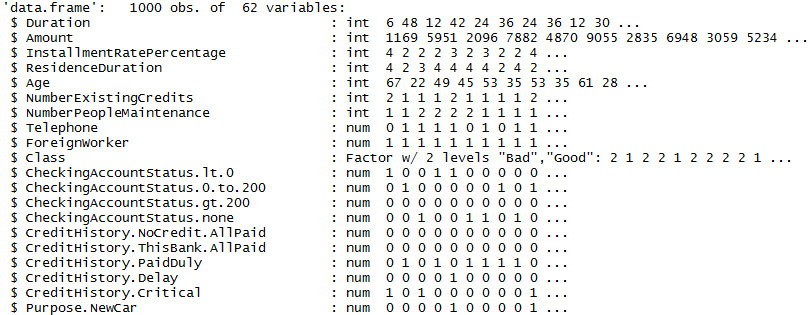###### Figure 1.17: A section of names in the GermanCredit dataset

The column name Telephone is of numeric data type. Few data values are also displayed alongside it to explain the column values.

5. The summary of the data can be obtained by the summary function:

# Display the summary of GermanCredit

summary(GermanCredit)

A section of the output is as follows: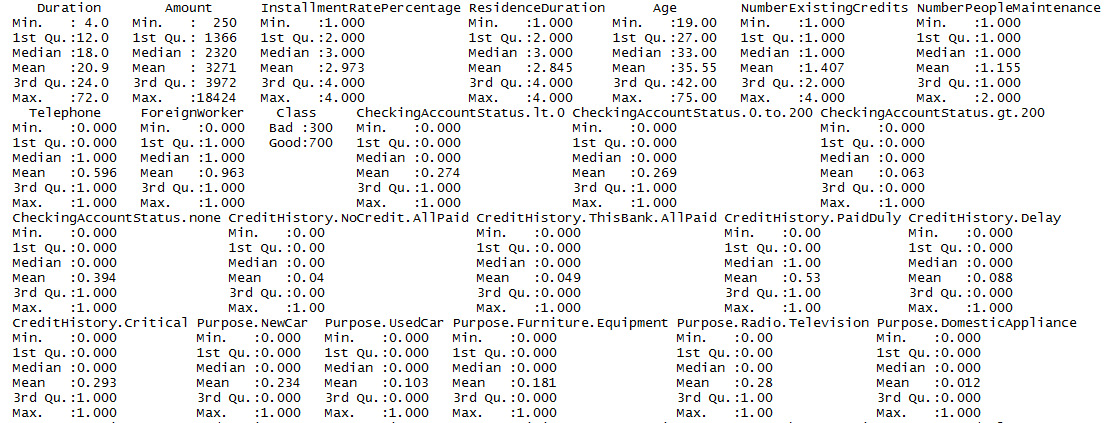###### Figure 1.18: A section of the summary of the GermanCredit dataset

The summary provides information such as minimum value, 1st quantile, median, mean, 3rd quantile, and maximum value. The description of these values is as follows: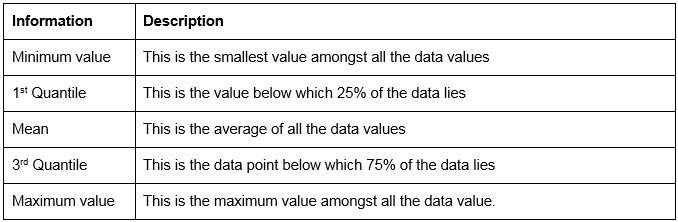###### Figure 1.19: Summary parameters
6. To view the summary of only one column, the particular column can be passed to the summary function:

# Display the summary of column 'Amount'

summary(GermanCredit\$Amount)

The output is as follows:

Min. 1st Qu.  Median    Mean 3rd Qu.    Max.

250    1366    2320    3271    3972   18424

We've had a glimpse of the data. Now, let's visualize it.

### Visualizing the Data

Data can be difficult to interpret. In this section, we will interpret it using graphs and other visualizing tools.

Histograms: A histogram displays the total count for each value of the column. We can view a histogram using the hist() function in R. The function requires the column name to be passed as the first parameter and the color of the bars displayed on the histogram as the second parameter. The name of the x axis is automatically given by the function as the column name:

#Histogram for InstallmentRatePercentage column

hist(GermanCredit\$InstallmentRatePercentage,col="red")

The output is as follows: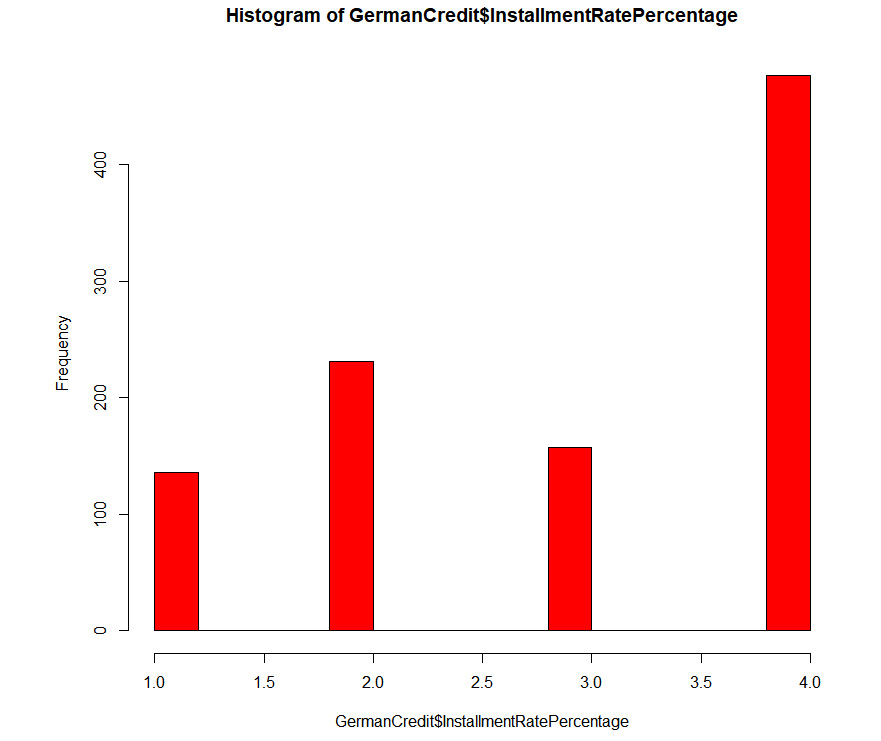###### Figure 1.20: An example histogram

Bar plots: Bar plots in the ggplot package are another way to visualize the count for a column of data. The aes() function allows color coding of the values. In the upcoming example, the number of gears is plotted against the count. We have color-coded the gear values using the aes() function. Now, the factor() function is used to display only the unique values on the axis. For instance, the data contains 3, 4, and 5, and so you will see only these values on the x axis.

# Bar Plots

ggplot(GermanCredit, aes(factor(ResidenceDuration),fill= factor(ResidenceDuration))) +geom_bar()

The output is as follows: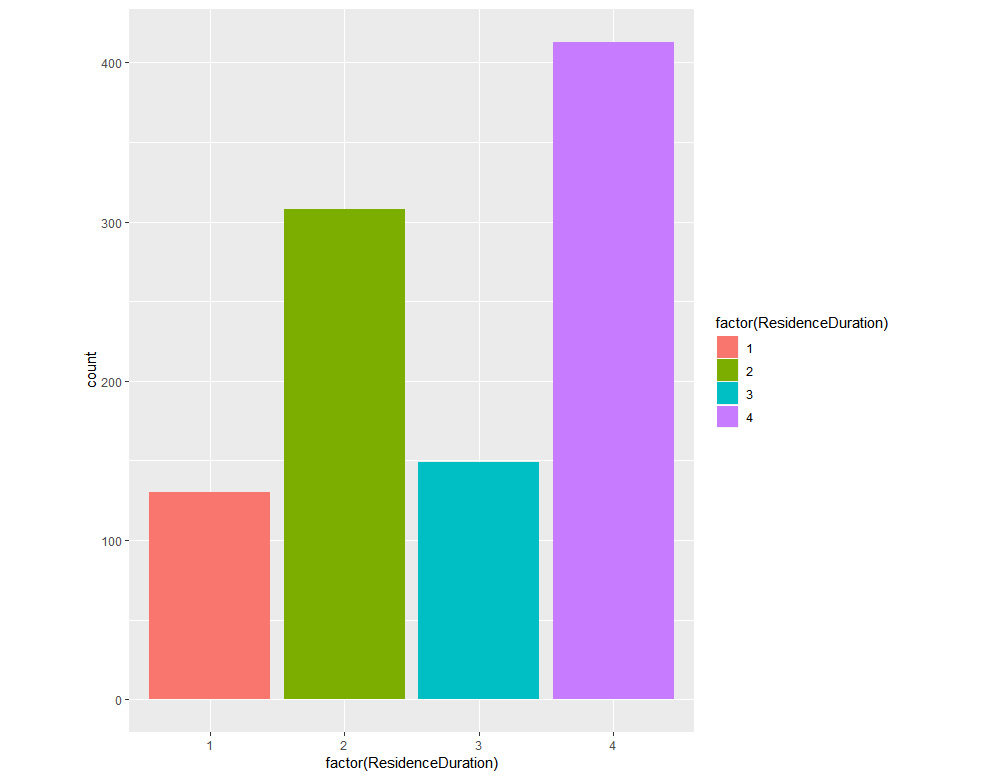###### Figure 1.21: An example bar plot

Scatter plots: This requires ggplot, which we installed in the previous exercises. We plot Age on the x axis, Duration on the y axis, and Class in the form of color.

install.packages("ggplot2",dependencies = TRUE)

#Scatter Plot

library(ggplot2)

qplot(Age, Duration, data = GermanCredit, colour =factor(Class))

The output is as follows: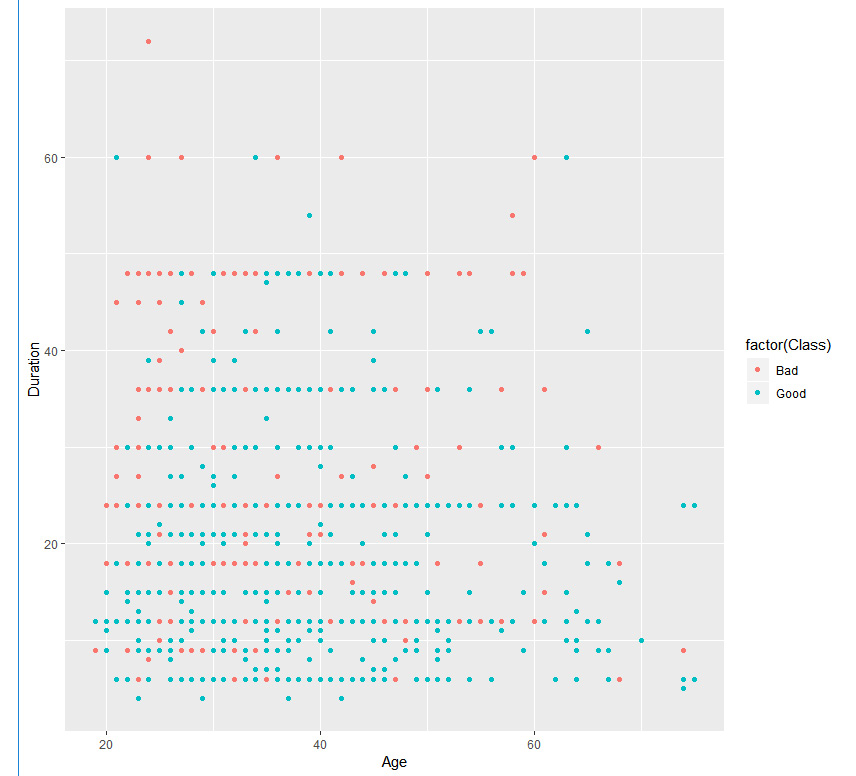###### Figure 1.22: An example scatter plot

We can also view the third column by adding the facet parameter, as shown here:

#Scatter Plot

library(ggplot2)

qplot(Age,Duration,data=GermanCredit,facets=Class~.,colour=factor(Class))

The output is as follows: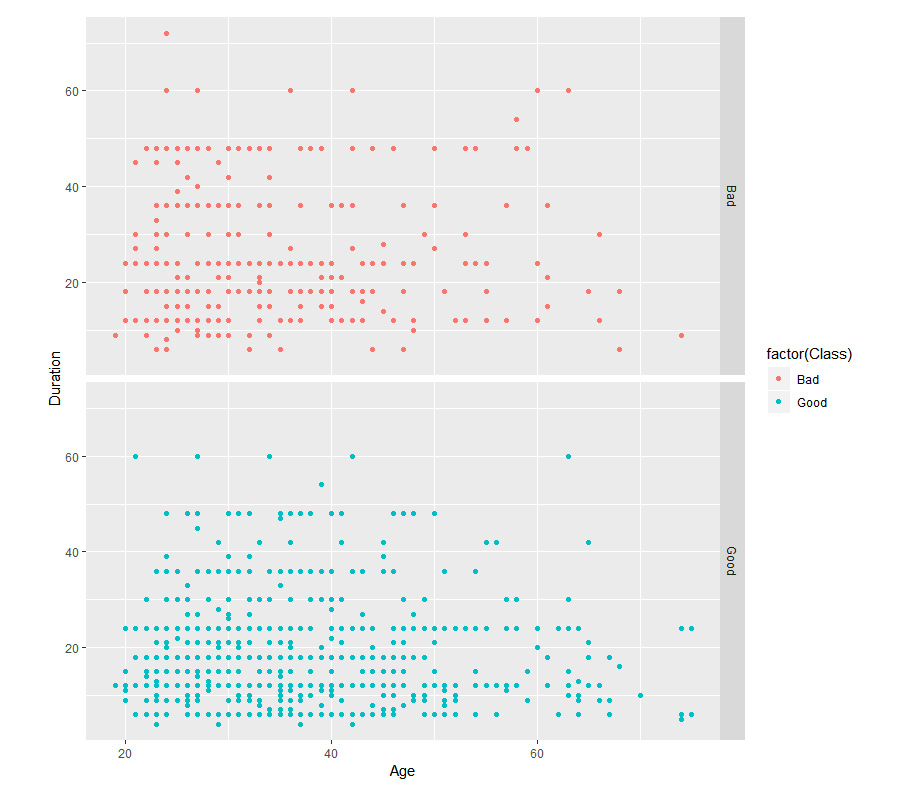###### Figure 1.23: An example scatter plot facet

Box Plots: We can view data distribution using a box plot. It shows the minimum, maximum, 1st quartile, and 3rd quartile. In R, we can plot it using the boxplot() function. The dataframe is provided to the data parameter. NumberExistingCredits is the y axis and InstallmentRatePercentage is the x axis. The name of the plot can be provided in main. The names for the x axis and y axis are given in xlab and ylab, respectively. The color of the boxes can be set using the col parameter. An example is as follows:

# Boxplot of InstallmentRatePercentage by Car NumberExistingCredits

boxplot(InstallmentRatePercentage~NumberExistingCredits,

data=GermanCredit, main="Sample Box Plot",

xlab="InstallmentRatePercentage",

ylab="NumberExistingCredits",

col="red")

The output is as follows: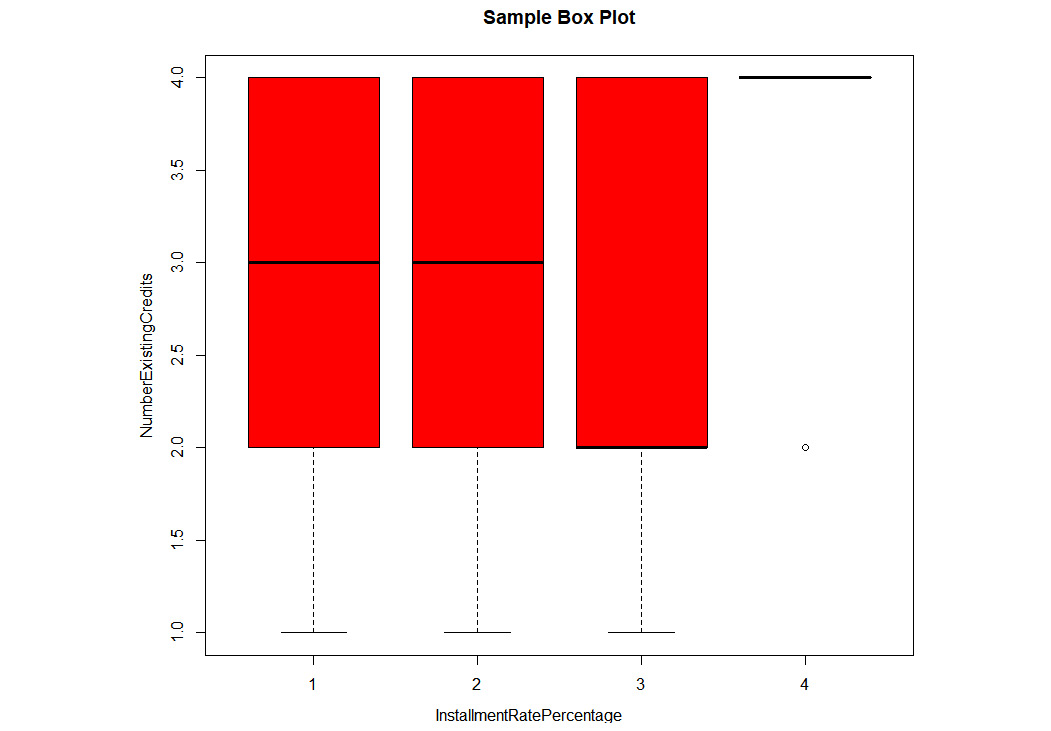###### Figure 1.24: An example box plot

Correlation: The correlation plot is used to identify the correlation between two features. The correlation value can range from -1 to 1. Values between (0.5, 1) and (-0.5, -1) mean strong positive correlation and strong negative correlation, respectively. The corrplot() function can plot the correlation of all the features with each other in a simple map. It is also known as a correlation heatmap:

#Plot a correlation plot

GermanCredit_Subset=GermanCredit[,1:9]

install.packages("corrplot")

library(corrplot)

correlations = cor(GermanCredit_Subset)

print(correlations)

The output is as follows: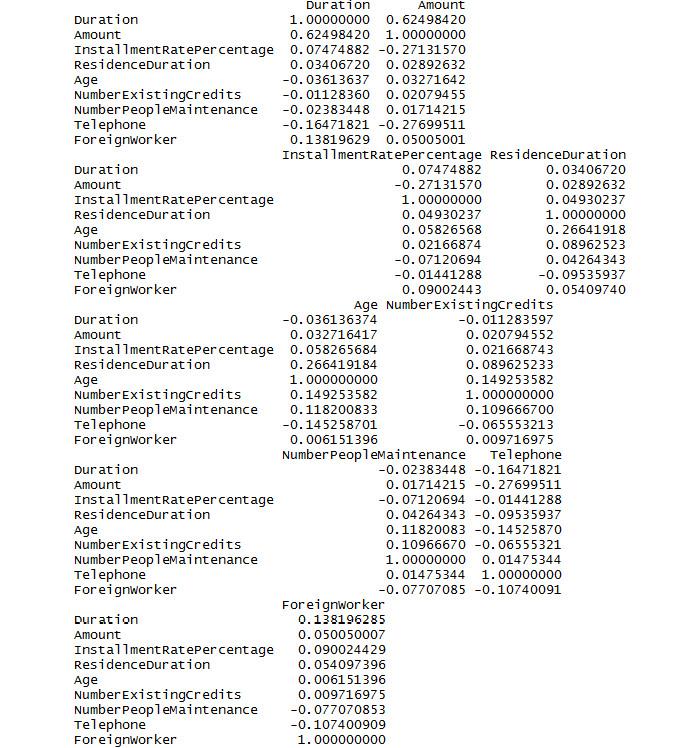###### Figure 1.25: A section of the output for correlations

The plot for correlations is as follows:

corrplot(correlations, method="color")

The output is as follows: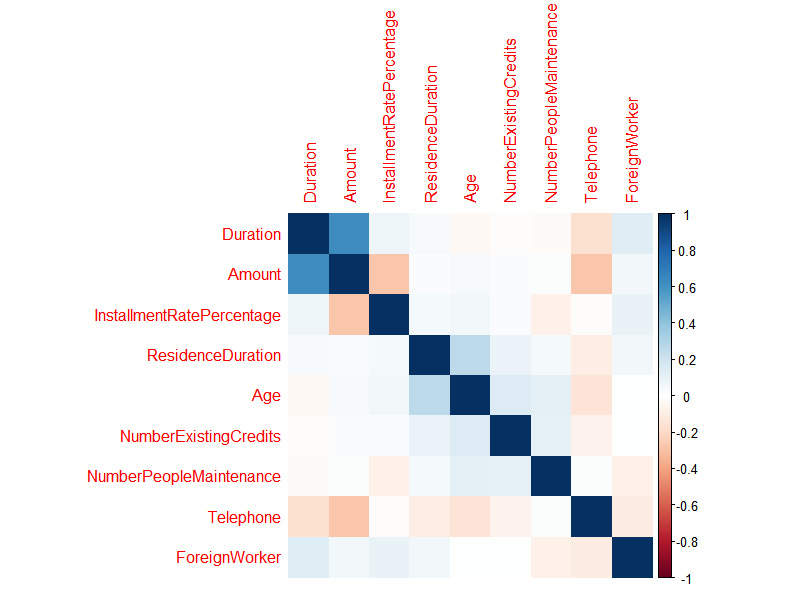###### Figure 1.26: A correlation plot

Density plot: The density plot can be used to view the distribution of the data. In this example, we are looking at the distribution of weight in the GermanCredit dataset:

#Density Plot

densityData <- density(GermanCredit\$Duration)

plot(densityData, main="Kernel Density of Weight")

polygon(densityData, col="yellow", border="green")

The output is as follows: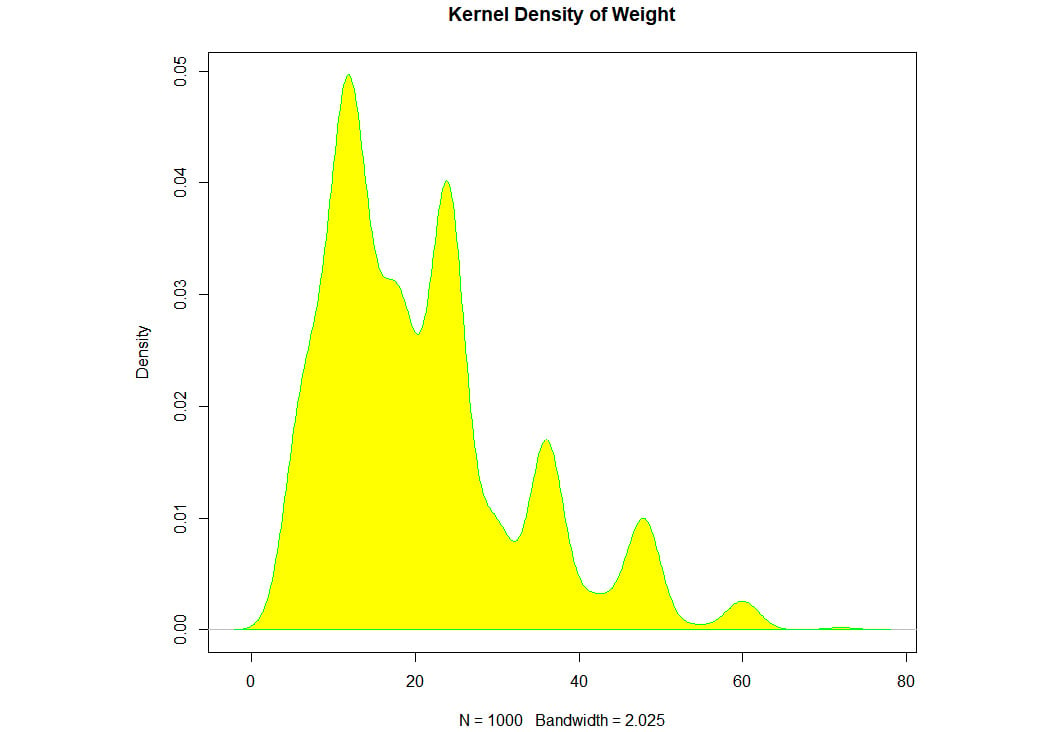###### Figure 1.27: An example density plot

We have learned about different plots. It's time to use them with a dataset.

### Activity 1: Finding the Distribution of Diabetic Patients in the PimaIndiansDiabetes Dataset

In this activity, we will load the PimaIndiansDiabetes dataset and find the age group of people with diabetes. The dataset can be found at https://github.com/TrainingByPackt/Practical-Machine-Learning-with-R/blob/master/Data/PimaIndiansDiabetes.csv.

The expected output should contain a bar plot of the count of positive and negative data present in the dataset with respect to age, as follows: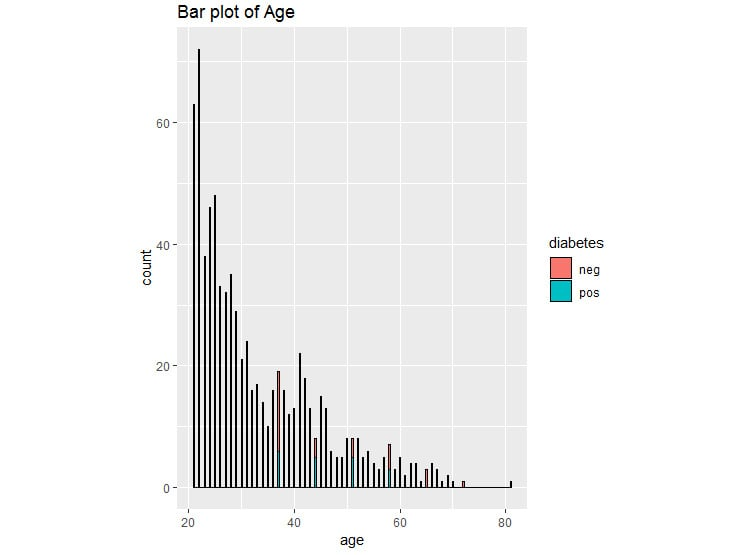###### Figure 1.28: Bar plot for diabetes

2. Create a PimaIndiansDiabetesData variable for further use.
3. View the first five rows using head().
4. Display the different unique values for the diabetes column.

#### Note

The solution for this activity can be found on page 312.

### Activity 2: Grouping the PimaIndiansDiabetes Data

During this activity, we will be viewing the summary of the PimaIndiansDiabetes dataset and grouping them to derive insights from the data.

1. Print the structure of the dataset. [Hint: use str()]
2. Print the summary of the dataset. [Hint: use summary()]
3. Display the statistics of the dataset grouped by diabetes column. [Hint: use describeBy(data,groupby)]

The output will show the descriptive statistics of the value of diabetes grouped by the pregnant value.

#Descriptive statistics grouped by pregnant values

Descriptive statistics by group

group: neg

vars   n  mean    sd median trimmed   mad min max range skew kurtosis   se

X1    1 500 68.18 18.06     70   69.97 11.86   0 122   122 -1.8     5.58 0.81

----------------------------------------------------------------------------------------------

group: pos

vars   n  mean    sd median trimmed   mad min max range  skew kurtosis   se

X1    1 268 70.82 21.49     74   73.99 11.86   0 114   114 -1.92     4.53 1.31

The output will show the descriptive statistics of the value of diabetes grouped by the pressure value.

#Descriptive statistics grouped by pressure values

Descriptive statistics by group

group: neg

vars   n mean   sd median trimmed  mad min max range skew kurtosis   se

X1    1 500  3.3 3.02      2    2.88 2.97   0  13    13 1.11     0.65 0.13

----------------------------------------------------------------------------------------------

group: pos

vars   n mean   sd median trimmed  mad min max range skew kurtosis   se

X1    1 268 4.87 3.74      4     4.6 4.45   0  17    17  0.5    -0.47 0.23

#### Note

The solution for this activity can be found on page 314.

### Activity 3: Performing EDA on the PimaIndiansDiabetes Dataset

During this activity, we will be plotting the correlation among the fields in the PimaIndiansDiabetes dataset so that we can find which of the fields have a correlation with each other. Also, we will create a box plot to view the distribution of the data so that we know the range of the data, and which data points are outliers. The dataset can be found at https://github.com/TrainingByPackt/Practical-Machine-Learning-with-R/blob/master/Data/PimaIndiansDiabetes.csv.

2. View the correlation among the features of the PimaIndiansDiabetes dataset.
3. Round it to the second nearest digit.
4. Plot the correlation.
5. Create a box plot to view the data distribution for the pregnant column and color by diabetes.

Once you complete the activity, you should obtain a boxplot of data distribution for the pregnant column, which is as follows: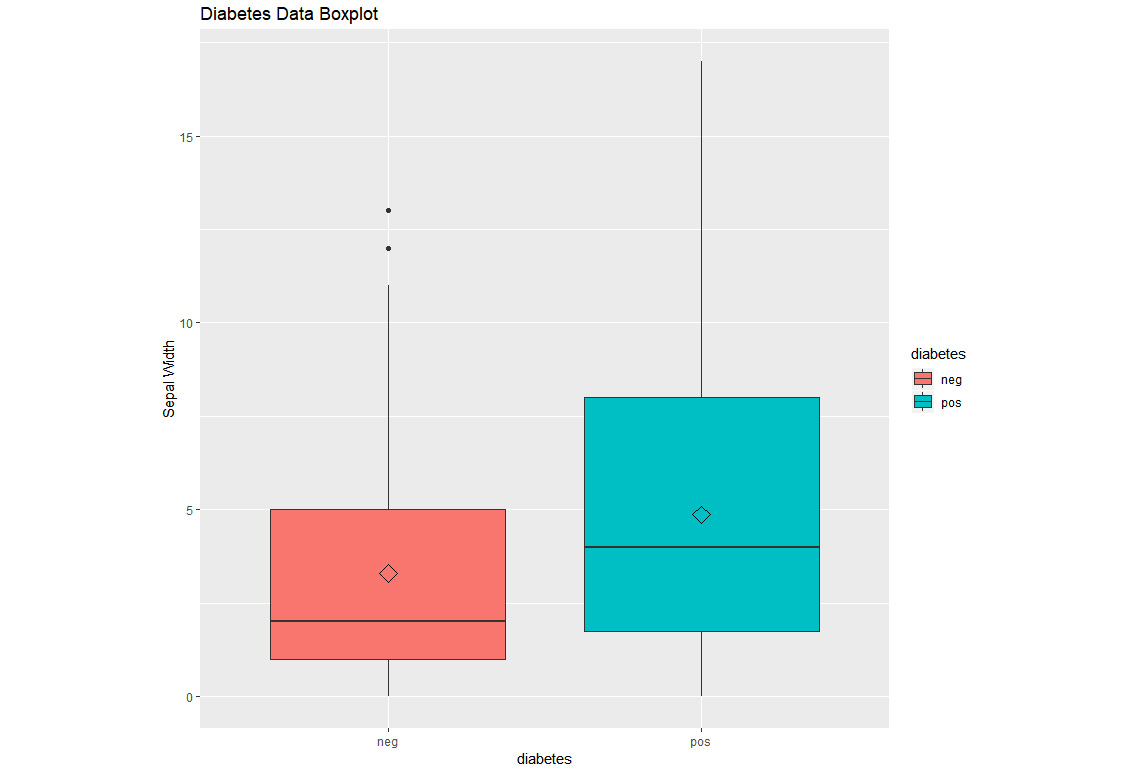#### Note

The solution for this activity can be found on page 316.

We have learned how to perform correlation among all the columns in a dataset and how to plot a box plot for individual fields and then color it by certain categorical values.

## Machine Learning Models

There are various algorithms that can be applied to numerous kinds of business problems. Broadly, the algorithms fall under supervised and unsupervised learning.

In supervised learning, the model is exposed to a set of samples with known outcomes/labels from which it learns. This process is known as training the model. After the learning/training process, the model is given a new set of data samples, based on which it performs predictions that give us the outcome. This is known as the prediction phase.

In unsupervised learning, the data samples provided to the model do not contain outcomes or labels. The model identifies patterns present in the samples and highlights the most commonly occurring patterns. Some of the approaches are clustering (hierarchical, k-means, and so on) and neural networks (self-organizing maps). This approach can also be used to detect unusual behavior in the data.

### Types of Prediction

The prediction techniques are broadly categorized into numeric prediction and categoric prediction.

Numeric prediction: When the output to be predicted is a number, it is called numeric prediction. As shown in the following example, the output is 5, 3.8, 7.6, which are numeric in nature: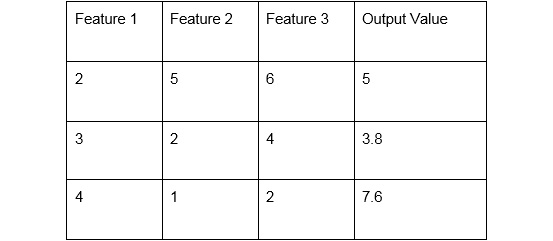###### Figure 1.30: Numeric prediction data

Categorical prediction: When the output to be predicted is a category (non-numeric), it is known as categorical prediction. It is mostly defined as a classification problem. The following data shows an example of categorical value prediction for the outputs A and G.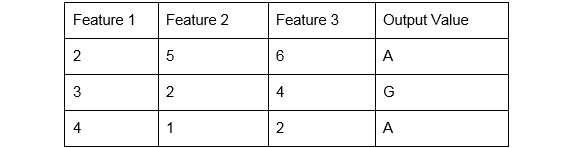###### Figure 1.31: Categorical prediction data

It is important to identify the nature of the output variable because the model should be chosen based on the type of output variable. In certain cases, the data is transformed into another type to cater to the requirements of the particular algorithm. We will now go through a list of machine learning algorithms in the following section and will discuss in detail the type of predictions they can be used for.

### Supervised Learning

Supervised learning is broadly classified as follows:

• Linear regression: This is a technique whereby the input variable and the output field are related by a linear equation, Y=aX+b. It can be implemented using the lm() function in R. This is used for predicting numerical values, for instance, predicting the revenue of a company for the next year.
• Logistic regression: This technique is used for a classification problem where the output is categorical in nature. For instance, will it rain tomorrow? The answer would be Y or N. This technique fits a function that is a closest fit of the data and is also a linear combination of the input variables. The glm() function in R is used for implementing it.
• Decision trees: A decision tree is a tree with multiple nodes and branches, where each node represents a feature and the branches from the node represent a condition for the feature value. The tree can have multiple branches, signifying multiple conditions. The leaf node of the tree is the outcome. If the outcome is continuous, it is known as a regression tree.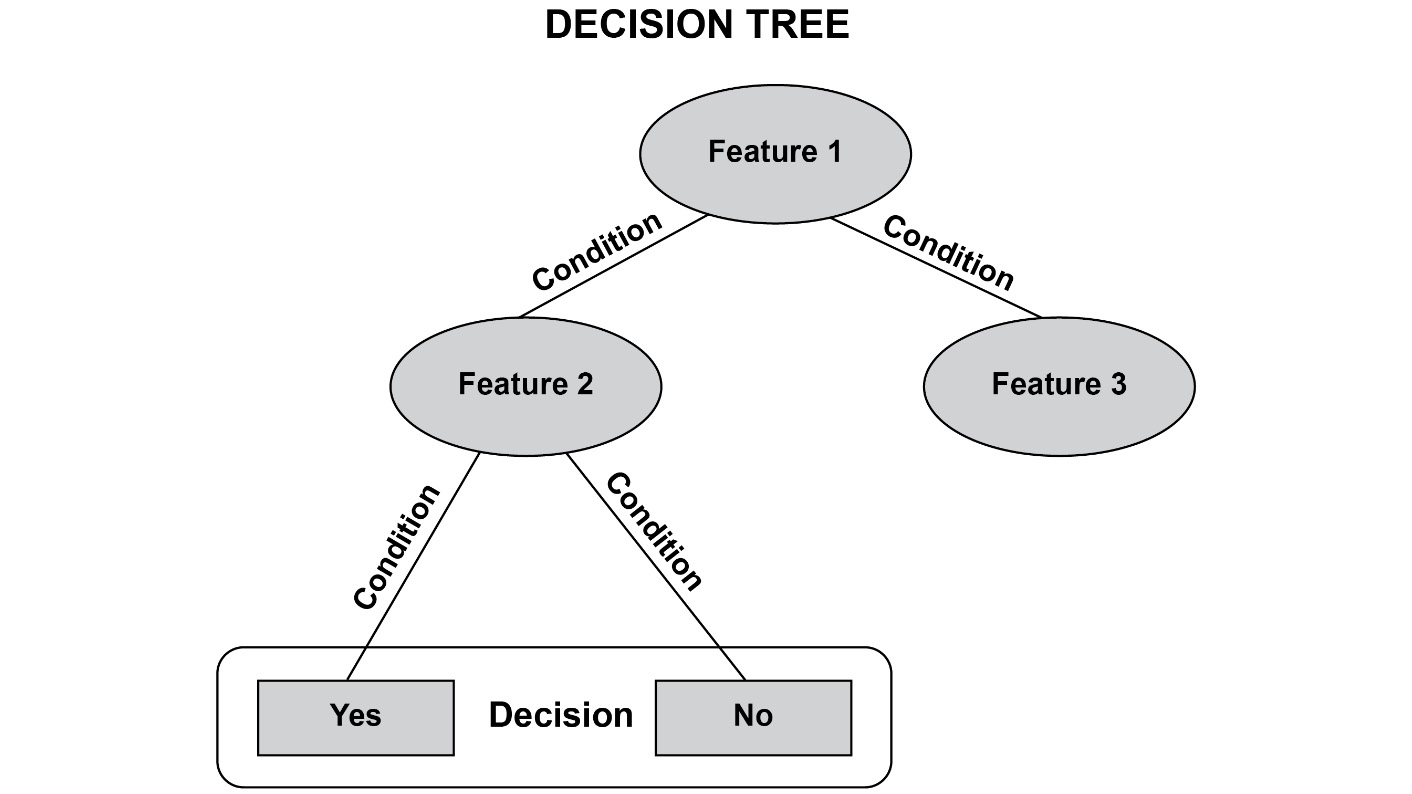###### Figure 1.32: A sample decision tree
• Support vector machines: This approach maps inputs to a higher dimensional space using a kernel function. The data is separated by hyperplanes in this higher dimensional space. The support vector machine identifies the most optimal hyperspace with a large separation. It can be used for classification problems such as categorical prediction, as well as numeric prediction. It is also known as support vector regression.
• Naïve Bayes: It is a probabilistic model that uses the Bayes' theorem to perform classification.
• Random forest: This can be used for performing classification and regression problems. It is an ensemble approach, which involves training multiple decision trees and combining the results to give the prediction.
• Neural networks: A neural network is inspired by the a human brain. It consists of interconnected neurons or nodes. It uses a backpropagation algorithm for learning. It can be used for categorical, as well as numeric, predictions.

### Unsupervised Learning

For unsupervised learning, hierarchical clustering and k-means clustering are used.

• Hierarchical clustering: The goal of a clustering process is to identify groups within the data. Each group must have data points that are very similar to each other. Also, two groups must be very different from each other in terms of their characteristics. Hierarchical clustering forms a dendrogram, a tree-like structure. There are two types of hierarchical clustering. Agglomerative clustering forms a tree-like structure in a bottom-up manner, whereas divisive hierarchical clustering forms a tree-like structure in a top-down manner.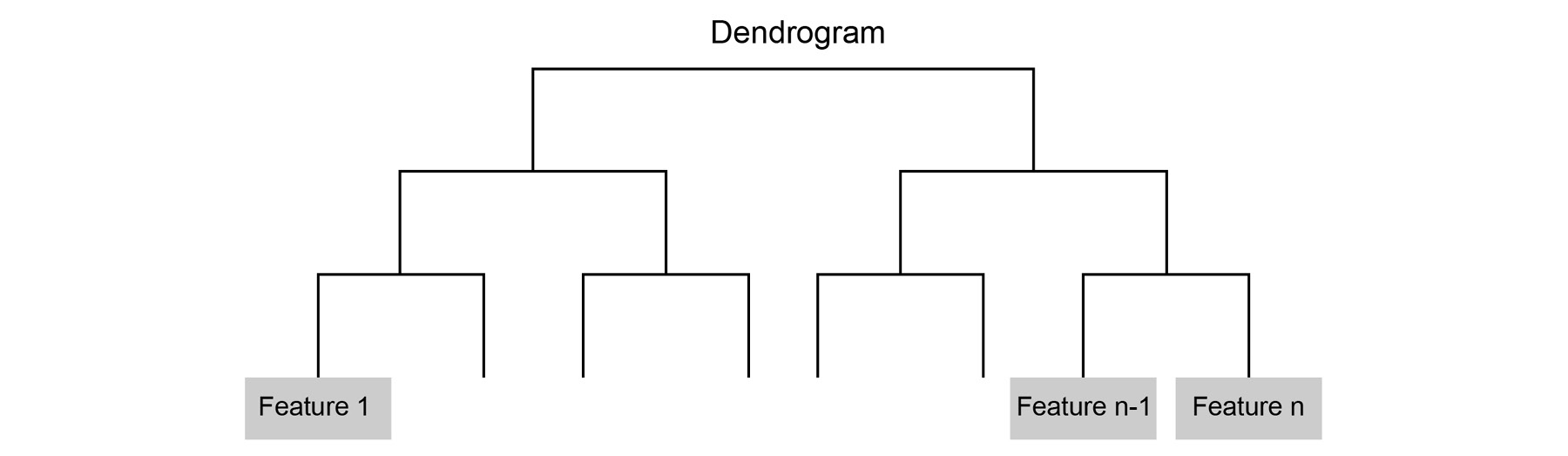###### Figure 1.33: A dendrogram
• K-means clustering: In k-means clustering, the data is grouped into k clusters. The data is grouped based on the similarity score between the data points. Here, k is a predefined value. The choice of the value determines the quality of the cluster.

### Applications of Machine Learning

The following are a few practical applications of machine learning:

• Recommendation systems: You can find a number of recommendations occurring on e-commerce websites. For instance, Amazon has several algorithms recommending products to its users. Predicting the user's next purchase or displaying products similar to the products purchased by the user are some scenarios where machine learning algorithms are used.
• Forecasting sales: In many product-based companies, the sales could be predicted for the next month/quarter/year. This can help them to better plan their resources and stocks.
• Fraud detection: Credit card fraud can be detected by using the transaction data. Classifiers can classify the fraudulent transactions, or outlier/anomaly detection can detect the anomalies in the transaction data.
• Sentiment analysis: Textual data is available in abundance. Sentiment analysis on textual data can be done using machine learning. For example, the user's sentiments can be identified as positive or negative, based on reviews of a certain product crawled from the internet. Logistic regression or Naïve Bayes can be used to identify the category using a bag of words representing the sentiments.
• Stock prediction: The stock price is predicted based on the characteristics of the stock in the past. Historical data containing the opening price, closing price, high, and low can be used.

## Regression

In this section, we will cover linear regression with single and multiple variables. Let's implement a linear regression model in R. We will predict the median value of an owner-occupied house in the Boston Housing dataset.

The Boston Housing dataset contains the following fields: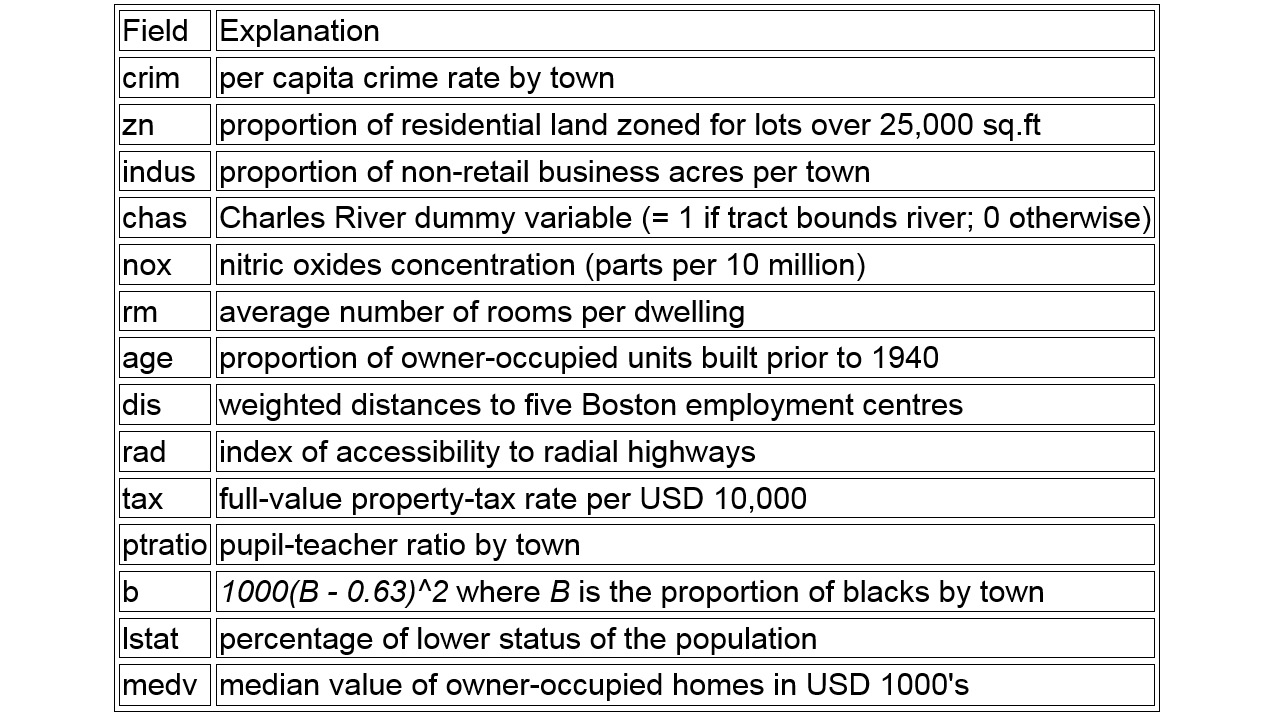###### Figure 1.34: Boston Housing dataset fields

Here is a model for the indus field.

#Build a simple linear regression

model1 <- lm(medv~indus, data = BostonHousing)

#summary(model1)

AIC(model1)

The output is as follows:

 3551.601

Build a model considering the age and dis fields:

model2 = lm(medv ~ age + dis, BostonHousing)

summary(model2)

AIC(model2)

Call:

lm(formula = medv ~ age + dis, data = BostonHousing)

Residuals:

Min      1Q  Median      3Q     Max

-15.661  -5.145  -1.900   2.173  31.114

Coefficients:

Estimate Std. Error t value Pr(>|t|)

(Intercept)  33.3982     2.2991  14.526  < 2e-16 ***

age          -0.1409     0.0203  -6.941  1.2e-11 ***

dis          -0.3170     0.2714  -1.168    0.243

---

Signif. codes:  0 ‘***’ 0.001 ‘**’ 0.01 ‘*’ 0.05 ‘.’ 0.1 ‘ ’ 1

Residual standard error: 8.524 on 503 degrees of freedom

Multiple R-squared:  0.1444,    Adjusted R-squared:  0.141

F-statistic: 42.45 on 2 and 503 DF,  p-value: < 2.2e-16

The output is as follows:

 3609.558

AIC is the Akaike information criterion, denoting that the lower the value, the better the model performance. Therefore, the performance of model1 is superior to that of model2.

In a linear regression, it is important to find the distance between the actual output values and the predicted values. To calculate RMSE, we will find the square root of the mean of the squared error using sqrt(sum(error^2)/n).

We have learned to build various regression models with single or multiple fields in the preceding example.

Another type of supervised learning is classification. In the next exercise we will build a simple linear classifier, to see how similar that process is to the fitting of linear regression models. After that, you will dive into building more regression models in the activities.

### Exercise 5: Building a Linear Classifier in R

In this exercise, we will build a linear classifier for the GermanCredit dataset using a linear discriminant analysis model.

The German Credit dataset contains the credit-worthiness of a customer (whether the customer is 'good' or 'bad' based on their credit history), account details, and so on. The dataset can be found at https://github.com/TrainingByPackt/Practical-Machine-Learning-with-R/blob/master/Data/GermanCredit.csv.

library(caret)

data(GermanCredit)

#OR

2. Subset the dataset:

#Subset the data

GermanCredit_Subset=GermanCredit[,1:10]

3. Find the fit model:

# fit model

fit <- lda(Class~., data=GermanCredit_Subset)

4. Summarize the fit:

# summarize the fit

summary(fit)

The output is as follows:

Length Class  Mode

prior    2     -none- numeric

counts   2     -none- numeric

means   18     -none- numeric

scaling  9     -none- numeric

lev      2     -none- character

svd      1     -none- numeric

N        1     -none- numeric

call     3     -none- call

terms    3     terms  call

xlevels  0     -none- list

5. Make predictions.

# make predictions

predictions <- predict(fit, GermanCredit_Subset[,1:10],allow.new.levels=TRUE)\$class

6. Calculate the accuracy of the model:

# summarize accuracy

accuracy <- mean(predictions == GermanCredit_Subset\$Class)

7. Print accuracy:

accuracy

The output is as follows:

 0.71

In this exercise, we have trained a linear classifier to predict the credit rating of customers with an accuracy of 71%. In chapter 4, Introduction to neuralnet and Evaluation Methods, we will try to beat that accuracy, and investigate whether 71% is actually a good accuracy for the given dataset.

### Activity 4: Building Linear Models for the GermanCredit Dataset

In this activity, we will implement a linear regression model on the GermanCredit dataset. The dataset can be found at https://github.com/TrainingByPackt/Practical-Machine-Learning-with-R/blob/master/Data/GermanCredit.csv.

2. Subset the data.
3. Fit a linear model for predicting Duration using lm().
4. Summarize the results.
5. Use predict() to predict the output variable in the subset.
6. Calculate Root Mean Squared Error.

Expected output: In this activity, we expect an RMSE value of 76.3849.

#### Note

The solution for this activity can be found on page 319.

In this activity, we have learned to build a linear model, make predictions on new data, and evaluate performance using RMSE.

### Activity 5: Using Multiple Variables for a Regression Model for the Boston Housing Dataset

In this activity, we will build a regression model and explore multiple variables from the dataset.

Refer to the example of linear regression performed with one variable and use multiple variables in this activity.

The dataset can be found at https://github.com/TrainingByPackt/Practical-Machine-Learning-with-R/blob/master/Data/BostonHousing.csv.

2. Build a regression model using multiple variables.
3. View the summary of the built regression model.
4. Plot the regression model using the plot() function.

The final graph for the regression model will look as follows: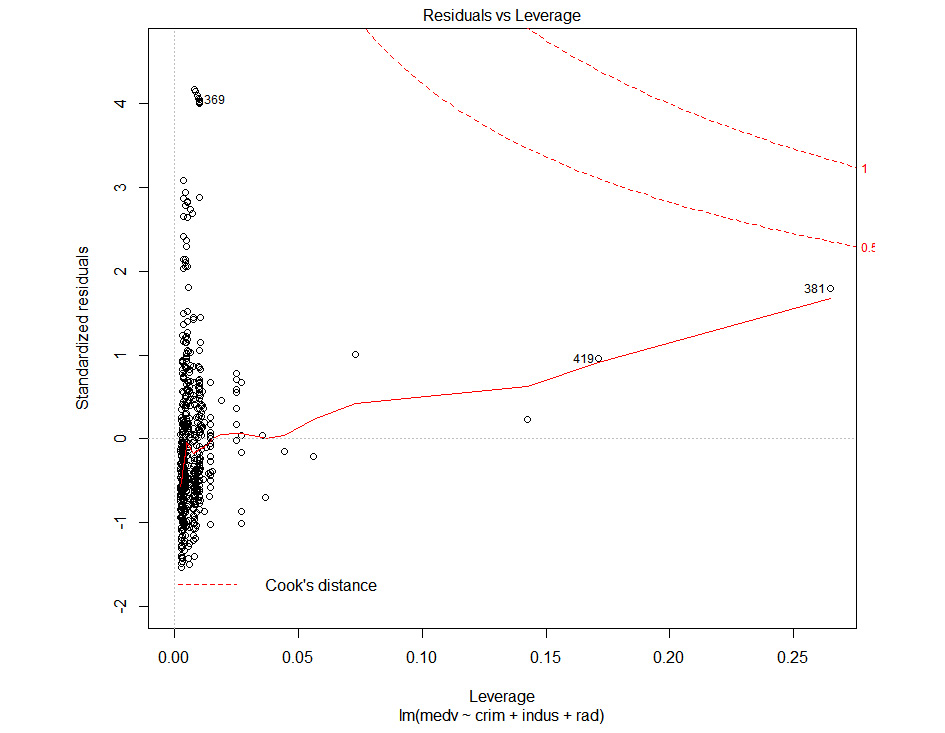#### Note

The solution for this activity can be found on page 320.

We have now explored the datasets with one or more variables.

## Summary

In this chapter, we learned about the machine learning process. The various steps are iterative in nature and ensure that the data is processed in a systematic manner. We explored the various evaluation metrics for evaluating a trained model. We also covered two types of variables: categorical and numeric.

We covered the different ways to view the summary of the data. We also delved into the various plots available in R for visualizing the data and for performing EDA. We looked at the German Credit, Boston Housing, and Diabetes datasets and performed some visualization on these datasets to understand them better. We also learned how to plot correlations for the features in the data and the ways to interpret them.

We looked into the common machine learning models used by data scientists. We also came to understand some of the types of models that can be used for numeric prediction and categorical prediction. Furthermore, we implemented a classifier in R and interpreted the results. We explored built-in datasets for building linear regression and classifier models.

Brindha Priyadarshini Jeyaraman is a senior data scientist at AIDA Technologies. She has completed her M.Tech in knowledge engineering with a gold medal from the National University of Singapore. She has more than 10 years of work experience and she is an expert in understanding business problems, and designing and implementing solutions using machine learning. She has worked on several real data science projects in the insurance and finance domain.

Browse publications by this author
• Ludvig Renbo Olsen

Ludvig Renbo Olsen, BSc in Cognitive Science from Aarhus University, is the author of multiple R packages, such as groupdata2 and cvms. With 4 years of R and Python experience, including working as a machine learning researcher at the Danish startup UNSILO, he is passionate about creating tools and tutorials for students and scientists. Guided by Effective Altruism, he intends to positively impact the world through his career.

Browse publications by this author
• Monicah Wambugu

Monicah Wambugu is the lead Data Scientist at Loanbee, a financial technology company that offers micro-loans by leveraging on data, machine learning and analytics to perform alternative credit scoring. She is a graduate student at the School of Information at UC Berkeley Masters in Information Management and Systems. Monicah is particularly interested in how data science and machine learning can be used to design products and applications that respond to the behavioral and socio-economic needs of target audiences.

Browse publications by this author